Function Repository Resource:

# GramP

Evaluate the Gram polynomial

Contributed by: Jan Mangaldan
 ResourceFunction["GramP"][k,m,t] gives the Gram polynomial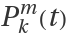.

## Details

Mathematical function, suitable for both symbolic and numerical manipulation.
Explicit formulas are given for nonnegative integer k.
For certain special arguments, ResourceFunction["GramP"] automatically evaluates to exact values.
ResourceFunction["GramP"] can be evaluated to arbitrary numerical precision.

## Examples

### Basic Examples (2)

Compute the fifth Gram polynomial:

 In:=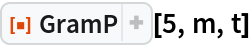Out=Plot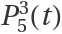over a subset of the reals:

 In:=Out=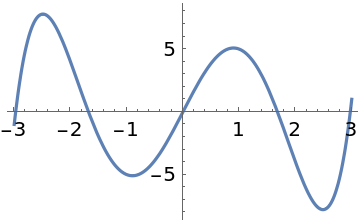### Scope (2)

Evaluate to high precision:

 In:=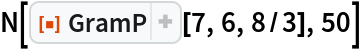Out=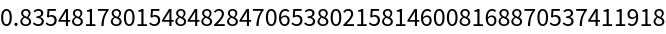In:=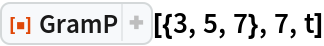Out=### Applications (2)

Plot the first few Gram polynomials:

 In:=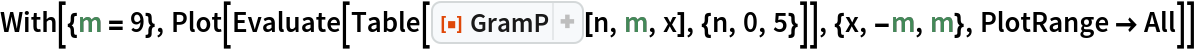Out=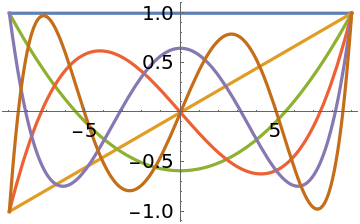Use Gram polynomials to construct a quartic least-squares fit for equispaced data:

 In:=Construct the basis functions and the design matrix:

 In:=The normal equations are easily solved in the Gram basis:

 In:=Out=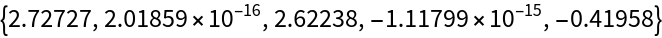Show the data with the curve:

 In:=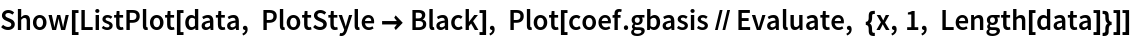Out=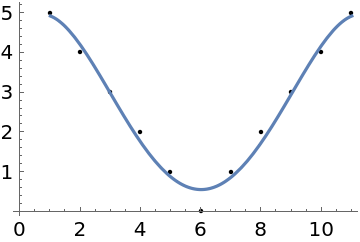### Properties and Relations (3)

GramP can be represented as a DifferenceRoot:

 In:=In:=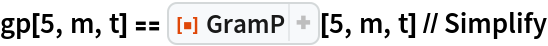Out=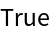GramP satisfies a discrete orthogonality relation:

 In:=Out=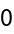The elements of SavitzkyGolayMatrix can be expressed in terms of GramP:

 In:=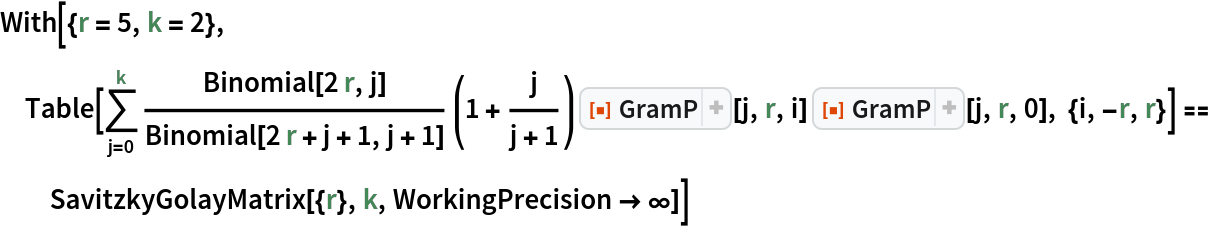Out=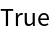In:=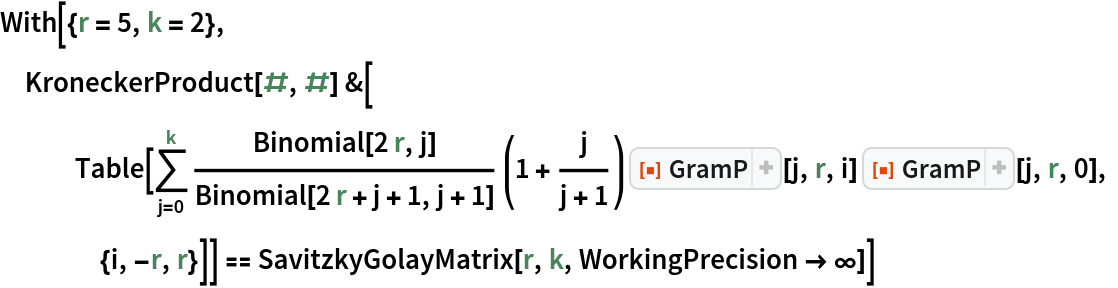Out=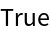## Version History

• 1.0.0 – 06 January 2021